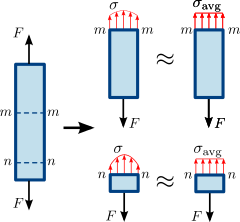## Stress Force Area Calculator

 I want to calculate Stress(σ) Force(F) Area(A) Force(F) = N Area(A) = m2 Stress(σ) = Pa

The ratio σ = F/A is an average stress### Stress Force Area formula:

Stress: σ＝F/A

where, A = Area, F = Force, σ = Stress.

For example, when area is 5, F is 2, then σ = 2/5 = 0.4 Pa.

Thinkcalculator.com provides you helpful and handy calculator resources.